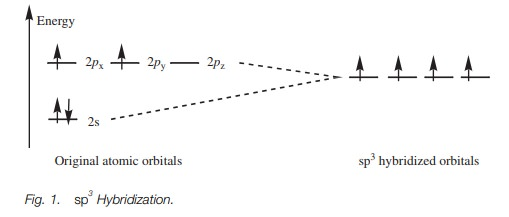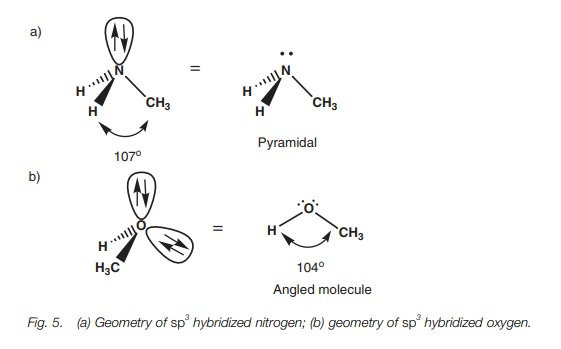Home | | Organic Chemistry | sp3 Hybridization

# sp3 Hybridization

Definition In sp3 hybridization, the s and the p orbitals of the second shell are ‘mixed’ to form four hybridized sp3 orbitals of equal energy.

SPHYBRIDIZATION

## Definition

In sp3 hybridization, the s and the p orbitals of the second shell are ‘mixed’ to form four hybridized sp3 orbitals of equal energy.

## Electronic configuration

Each hybridized orbital contains a single unpaired electron and so four bonds are possible.

## Geometry

Each sp3 orbital is shaped like a deformed dumbbell with one lobe much larger than the other. The hybridized orbitals arrange themselves as far apart from each other as possible such that the major lobes point to the cor-ners of a tetrahedron. sp3 Hybridization explains the tetrahedral carbon in saturated hydrocarbon structures.

## Sigma bonds

Sigma (σ) bonds are strong bonds formed between two sp3 hybridized car-bons or between ansp3 hybridized carbon and a hydrogen atom. Aσ bond formed between two sp3 hybridized carbon atoms involves the overlap of half filled sp3 hybridized orbitals from each carbon atom. Aσ bond formed between an sp3 hybridized carbon and a hydrogen atom involves a half-filled sp3 orbital from carbon and a half-filled 1s orbital from hydrogen.

## Nitrogen, oxygen and chlorine

Nitrogen, oxygen, and chlorine atoms can also be sp3 hybridized in organic molecules. This means that nitrogen has three half-filled sp3 orbitals and can form three bonds which are pyramidal in shape. Oxygen has two half-filled sp3orbitals and can form two bonds which are angled with respect to eachother. Chlorine has a single half-filled sp3 orbital and can only form a single bond. All the bonds which are formed are σ bonds.

## Definition

In sp3 hybridization, the 2s orbital is mixed with all three of the 2p orbitals to give a set of four sp3  hybrid orbitals. (The number of hybrid orbitals must equal the number of original atomic orbitals used for mixing.) The hybrid orbitals will each  have  the  same  energy  but  will  be  different  in  energy  from  the  original atomic  orbitals.  That  energy  difference  will  reflect  the  mixing  of  the  respect- ive atomic orbitals.  The energy of each hybrid orbital is greater than the original s orbital but less than the original p orbitals (Fig. 1).## Electronic configuration

The valence electrons for carbon can now be fitted into the sp3 hybridized orbitals (Fig. 1). There was a total of four electrons in the original 2s and 2p orbitals. The s orbital was filled and two of the p orbitals were half filled. After hybridization,there is a total of four hybridized sp3  orbitals all of equal energy. By Hund’s rule, they are all half filled with electrons which means that there are four unpaired electrons. Four bonds are now possible.

## Geometry

Each of the sp3 hybridized orbitals has the same shape – a rather deformed looking dumbbell (Fig. 2).  This deformed dumbbell looks more like a p orbital than an s orbital since more p orbitals were involved in the mixing process.Each sp3 orbital will occupy a space as far apart from each other as possible by pointing to the corners of a tetrahedron (Fig. 3). Here, only the major lobe of each hybridized orbital has been shown and the angle between each of these lobes is 109.5 . This is what is meant by the expression tetrahedral carbon. The three-dimensional shape of the tetrahedral carbon can be represented by drawing a nor-mal line for bonds in the plane of the page. Bonds going behind the page are represented by a hatched wedge, and bonds coming out the page are represented by a solid wedge.## Sigma bonds

A half-filled sp3 hybridized orbital from one carbon atom can be used to form a bond with a half-filled sp3 hybridized orbital from another carbon atom. In Fig. 4a, the major lobes of the two sp3 orbitals overlap directly leading to a strong σ bond. It is the ability of hybridized orbitals to form strong σ bonds that explains why hybridization takes place in the first place. The deformed dumbbell shapes allow a much better orbital overlap than would be obtained from a pure s orbital or a pure p orbital. A σ bond between an sp3 hybridized carbon atom and a hydrogen atom involves the carbon atom using one of its half-filled sp3 orbitals and the hydrogen atom using its half-filled 1s orbital (Fig. 4b).## Nitrogen, oxygen, and chlorine

Nitrogen,  oxygen  and  chlorine  atoms  can  also  be  sp3   hybridized  in  organic structures. Nitrogen has five valence electrons in its second shell. After hybridization, it will have three half-filled sp3 orbitals and can form three bonds. Oxygen has six valence electrons. After hybridization, it will have two half-filled sp3 orbitals and will form two bonds. Chlorine has seven valence electrons. After hybridization, it will have one half-filled sp3 orbital and will form one bond.

The four sp3 orbitals for these three atoms form a tetrahedral arrangement with one or more of the orbitals occupied by a lone pair of electrons. Considering the atoms alone, nitrogen forms a pyramidal shape where the bond angles are slightly less than 109.5° (c. 107°) (Fig. 5a). This compression of the bond angles is due to the orbital containing the lone pair of electrons, which demands a slightly greater amount of space than a bond. Oxygen forms an angled or bent shape where two lone pairs of electrons compress the bond angle from 109.5° to c. 104 (Fig. 5b).Alcohols, amines, alkyl halides, and ethers all contain sigma bonds involving nitrogen, oxygen, or chlorine. Bonds between these atoms and carbon are formed by the overlap of half-filled sp3 hybridized orbitals from each atom. Bonds involv-ing hydrogen atoms (e.g. O–H and N–H) are formed by the overlap of the half-filled 1s orbital from hydrogen and a half-filled sp3 orbital from oxygen or nitrogen.

Study Material, Lecturing Notes, Assignment, Reference, Wiki description explanation, brief detail
Organic Chemistry: Structure and bonding : sp3 Hybridization |